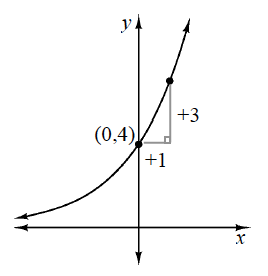### Home > AC > Chapter 14 > Lesson 14.3.1.5 > Problem3-64

3-64.

Use the clues in the graph at right to find a possible corresponding equation in $y=ab^x$ form. Assume the graph has an asymptote at the $x$-axis.

Use the information on the graph to find the coordinates of the unlabeled point.

The two points on the graph are $\left(0, 4\right)$ and $\left(1, 7\right)$.

What is the multiplier, or common ratio?

$y = 4\left(1.75\right)^{x}$